## Perimeter calculator for 2D Shapes

#### Perimeter Calculator

Result:

 Perimeter(Circumference) of Circle: 0

Result:

 Perimeter of Rectangle: 0

Result:

 Perimeter of Square: 0

Result:

 Perimeter of Triangle: 0

Result:

 Perimeter of Sector: 0

Result:

 Perimeter of Ellipse: 0

Result:

 Perimeter of Parallelogram: 0

### What is a perimeter?

A perimeter is defined as the total distance that surrounds a two-dimensional figure. This term is used for a path or its length. It is essentially the distance described by the outline of a shape. The perimeter for a circle is called its circumference.

### Perimeter Formulas

Below are the formulas to calculate the perimeters of different two-dimensional shapes.

#### Circle

A circle is a closed curve in which all the points on its boundary are at a fixed distance from a given point, which is designated the centre of the circle.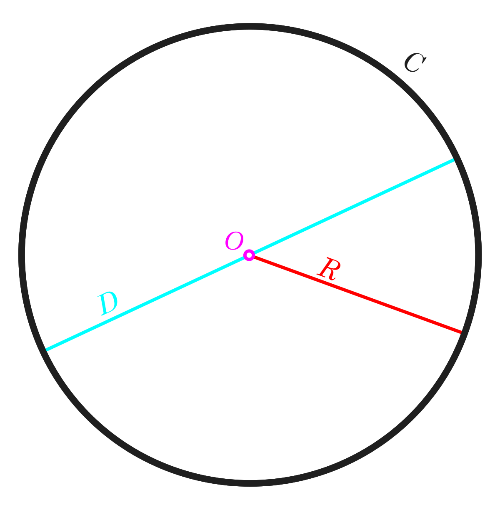In figure above, O indicates the centre of the circle. The distance between the centre and all the points on the circumference (or the boundary) of the circle is called the radius, marked with R.

The perimeter of a circle is called its circumference, the formula for which is 2πR units.

#### Rectangle

A rectangle is a polygon having four sides and four vertices, and the angle at each vertex is a right angle. The opposite sides of a rectangle are equal in length and parallel to each other. A rectangle has a specific length and width, which are different from each other.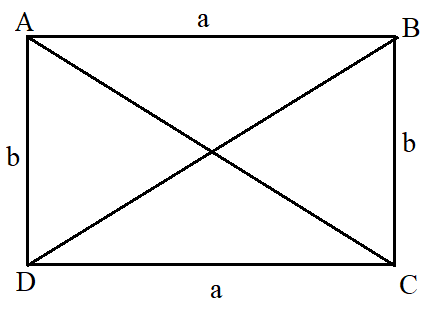In this figure, the sides AB = CD = a (length of the rectangle), and AD = BC = b (width). The perimeter is calculated as 2 (a + b) units.

#### Square

A square is a special case of a rectangle in which all the sides are equal in length. Each interior angle measures 90⁰, and the diagonals are also equal in length.The figure above shows a square where the length of the side is ‘a’ units. Hence, the perimeter is 4a units.

#### Triangle

A triangle is a polygon having three vertices and three edges. Depending on their sides, triangles are of three types.

• An equilateral triangle is one which all the sides are equal in length.
• An isosceles triangle has exactly two sides equal in length.
• A scalene triangle is a triangle where the three sides are of different lengths.

The figure below describes the three types of triangles.

.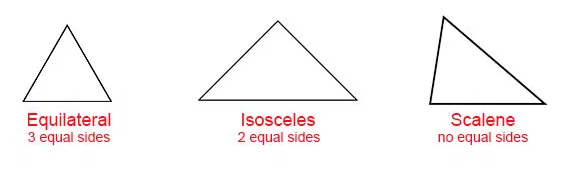The formula for the perimeter of a triangle is a + b + c units, where a, b and c indicate the lengths of the three sides.

#### Sector

A sector is the region of a circle bounded by two radii of the circle, and the arc in between. The arc is a small part of the circumference, and the radii are the endpoints of this arc. It is like a ‘pizza slice’ of the circle.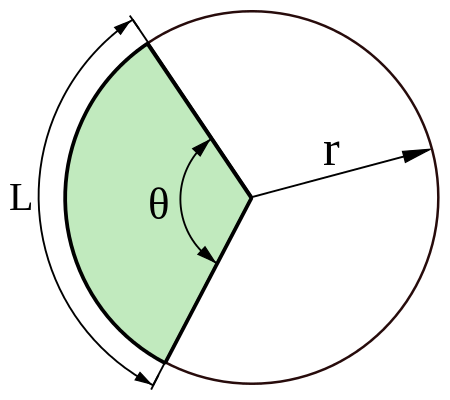Given above is the figure of a sector. In this figure, θ denotes the angle made by the sector at the centre of the circle, r is the radius and L is the arc length. The perimeter of a sector is the sum of the arc length and the two radii.

The formula is $$2r \;+ \; {(2πr \; {θ\over360})} \;units.$$

#### Ellipse

An ellipse is the set of all points in a plane such that the sum of their distances from two fixed points in the plane is a constant. These two fixed points are known as the foci of the ellipse. Given below is a figure of an ellipse.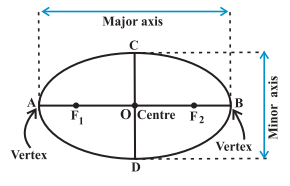In this figure, the two foci are F1 and F2. The ellipse is defined by its axes. The major axis of the ellipse is the longest diameter of the ellipse, and the minor axis is the smallest diameter. In the figure above, AB is the major axis, which is usually denoted by ‘a’ and CD is the minor axis, denoted by ‘b’.

The perimeter of an ellipse is $$2π \, \sqrt{{a^2+b^2}\over2} \; units$$

#### Parallelogram

A parallelogram is a polygon having four sides and four vertices, and in which the opposite sides are parallel to each other and equal in length. This has been shown in the figure below.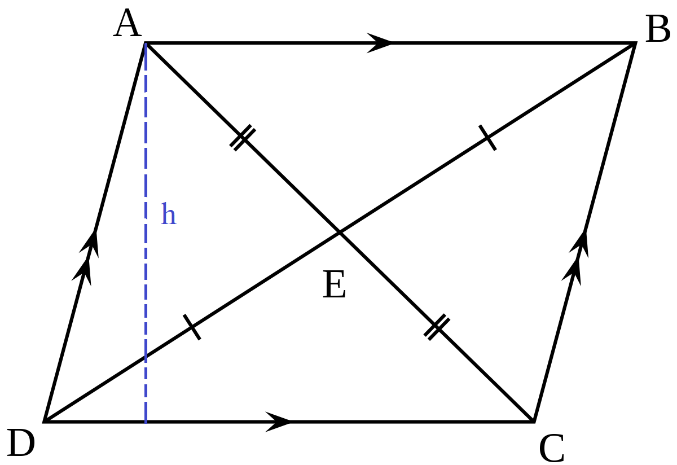ABCD is a parallelogram where the sides AB = CD = b, and AD = BC = a. Moreover, AB is parallel to CD, and AD is parallel to BC. The height h has been marked with a blue line.

The perimeter is calculated as 2 (a + b) units.

### Areas of application

Calculation of the perimeter has many real-life applications. For example, toa find the length of a fence surrounding a lawn, we need to know the perimeter of the lawn first. The perimeter/ circumference of a circle tells us how much distance the circle will cover in one revolution. We also need to find the perimeter of the string of Christmas lights when decorating the house. For finding the correct length of crochet or ribbon required to cover the border of a mat, we need to know the perimeter of the mat.